# SAT Math Multiple Choice Question 28: Answer and Explanation

### Test Information

Question: 28

4. If c =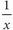+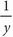and x > y > 0, then which of the following is equal to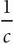?

• A. x + y
• B. x - y
• C.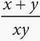• D.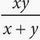D Here's yet another chance to plug in because of the variables in the answer choices. In this case, you have several variables. You should start by plugging in values for x and y, and then work out c. Because x > y > 0, let's say x = 6 and y = 3. Therefore, c =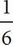+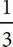=+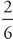=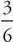=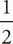. The question asks for the value of, which is the reciprocal of, or 2. This is your target answer. If you plug x = 6 and y = 3 into all of the answer choices, you'll find that only (D) equals 2.